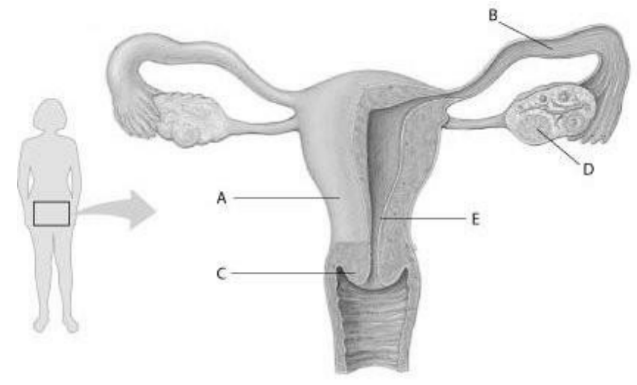# Problem: Refer to the following figure, which diagrams the reproductive anatomy of the human female, to answer the question.In the figure, which structure is the site of embryo implantation?a. Bb. Cc. Dd. E

🤓 Based on our data, we think this question is relevant for Professor Ozga's class at NSU.

###### Problem Details

Refer to the following figure, which diagrams the reproductive anatomy of the human female, to answer the question.

In the figure, which structure is the site of embryo implantation?

a. B

b. C

c. D

d. EWhat scientific concept do you need to know in order to solve this problem?

Our tutors have indicated that to solve this problem you will need to apply the Animal Reproduction concept. You can view video lessons to learn Animal Reproduction. Or if you need more Animal Reproduction practice, you can also practice Animal Reproduction practice problems.

What is the difficulty of this problem?

Our tutors rated the difficulty ofRefer to the following figure, which diagrams the reproducti...as medium difficulty.

How long does this problem take to solve?

Our expert Biology tutor, Kaitlyn took 5 minutes and 19 seconds to solve this problem. You can follow their steps in the video explanation above.

What professor is this problem relevant for?

Based on our data, we think this problem is relevant for Professor Ozga's class at NSU.